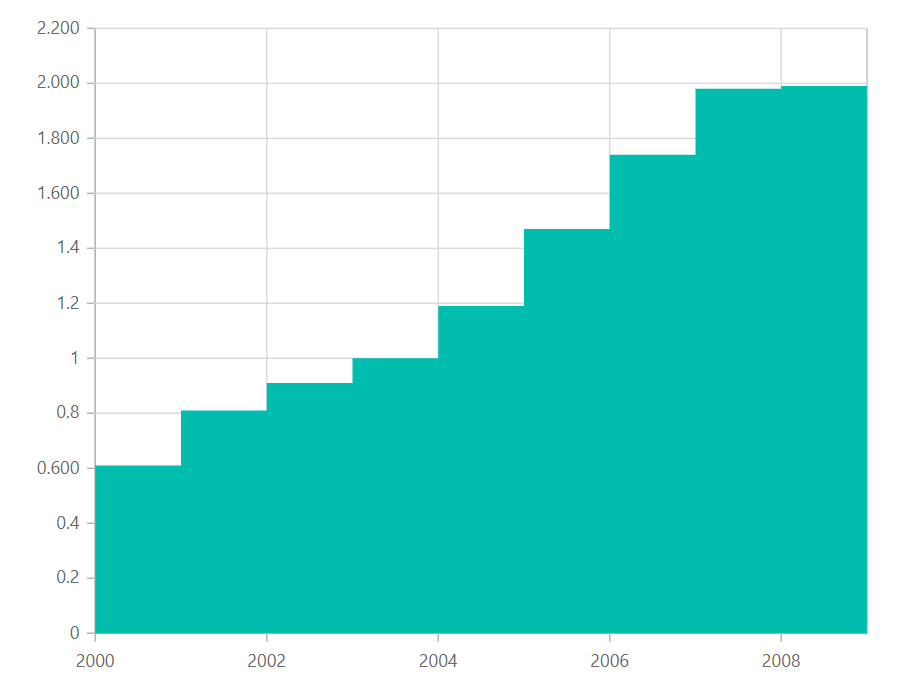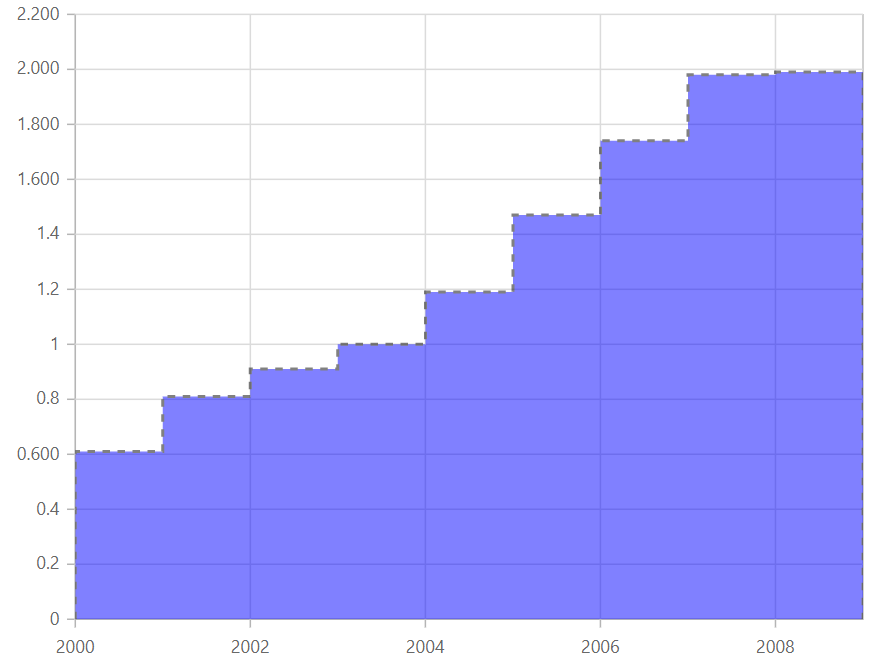Search results

# Step Area in Blazor Chart component

## Step Area

Like the step line chart, but with the areas connected with lines shaded. To render a step area series, use series `Type` as `StepArea`.

steparea.razor
``````@using Syncfusion.Blazor.Charts

<SfChart Width="60%">
<ChartSeriesCollection>
<ChartSeries DataSource="@WeatherReports" XName="X" YName="Y" Type="ChartSeriesType.StepArea">
</ChartSeries>
</ChartSeriesCollection>
</SfChart>
@code{
public class ChartData
{
public string X;
public double Low;
public double High;
}

public List<ChartData> WeatherReports = new List<ChartData>
{
new ChartData { X= "Sun", Low= 2.5, High= 9.8 },
new ChartData { X= "Mon", Low= 4.7, High= 11.4 },
new ChartData { X= "Tue", Low= 6.4, High= 14.4 },
new ChartData { X= "Wed", Low= 9.6, High= 17.2 },
new ChartData { X= "Thu", Low= 7.5, High= 15.1 },
new ChartData { X= "Fri", Low= 3.0, High= 10.5 },
new ChartData { X= "Sat", Low= 1.2, High= 7.9 }
};
}``````## Customization

You can use the following properties to customize the step area series.

``````@using Syncfusion.Blazor.Charts

<SfChart Width="60%">
<ChartSeriesCollection>
<ChartSeries DataSource="@WeatherReports" DashArray="5,5" Opacity="0.5" Fill="blue" XName="X" YName="Y" Type="ChartSeriesType.StepArea">
<ChartSeriesBorder Width="2" Color="black"></ChartSeriesBorder>
</ChartSeries>
</ChartSeriesCollection>
</SfChart>
@code{
public class ChartData
{
public string X;
public double Low;
public double High;
}

public List<ChartData> WeatherReports = new List<ChartData>
{
new ChartData { X= "Sun", Low= 2.5, High= 9.8 },
new ChartData { X= "Mon", Low= 4.7, High= 11.4 },
new ChartData { X= "Tue", Low= 6.4, High= 14.4 },
new ChartData { X= "Wed", Low= 9.6, High= 17.2 },
new ChartData { X= "Thu", Low= 7.5, High= 15.1 },
new ChartData { X= "Fri", Low= 3.0, High= 10.5 },
new ChartData { X= "Sat", Low= 1.2, High= 7.9 }
};
}``````